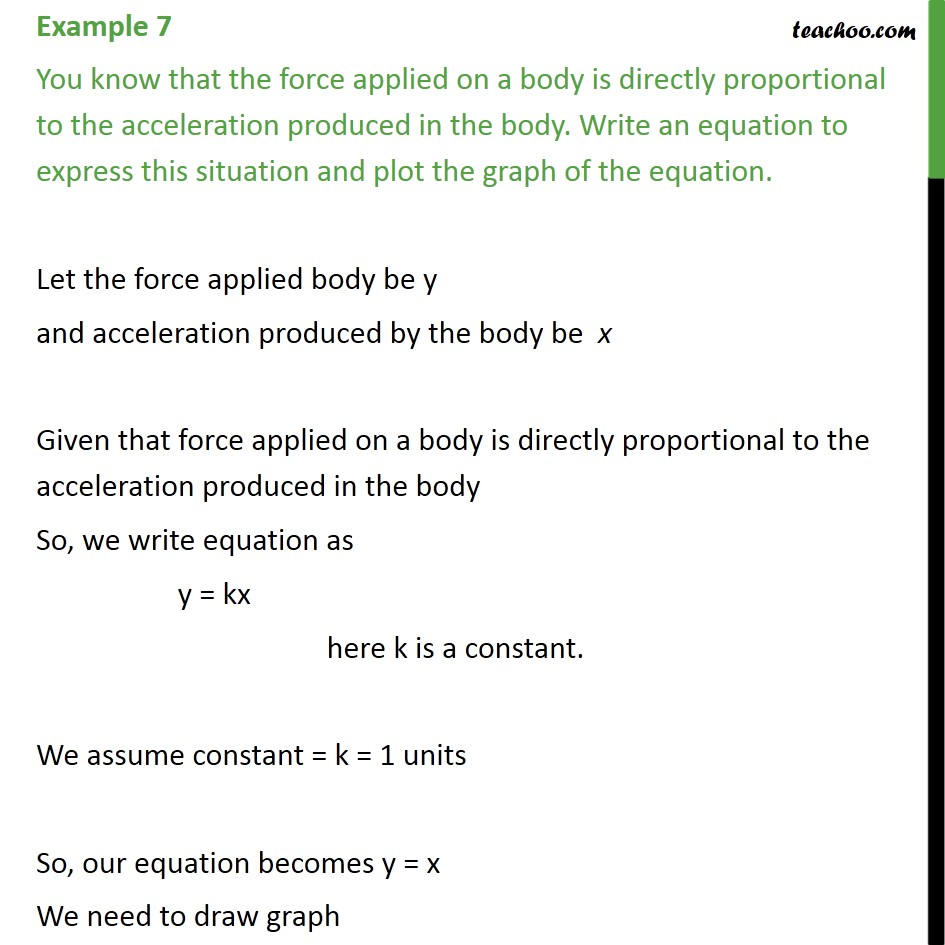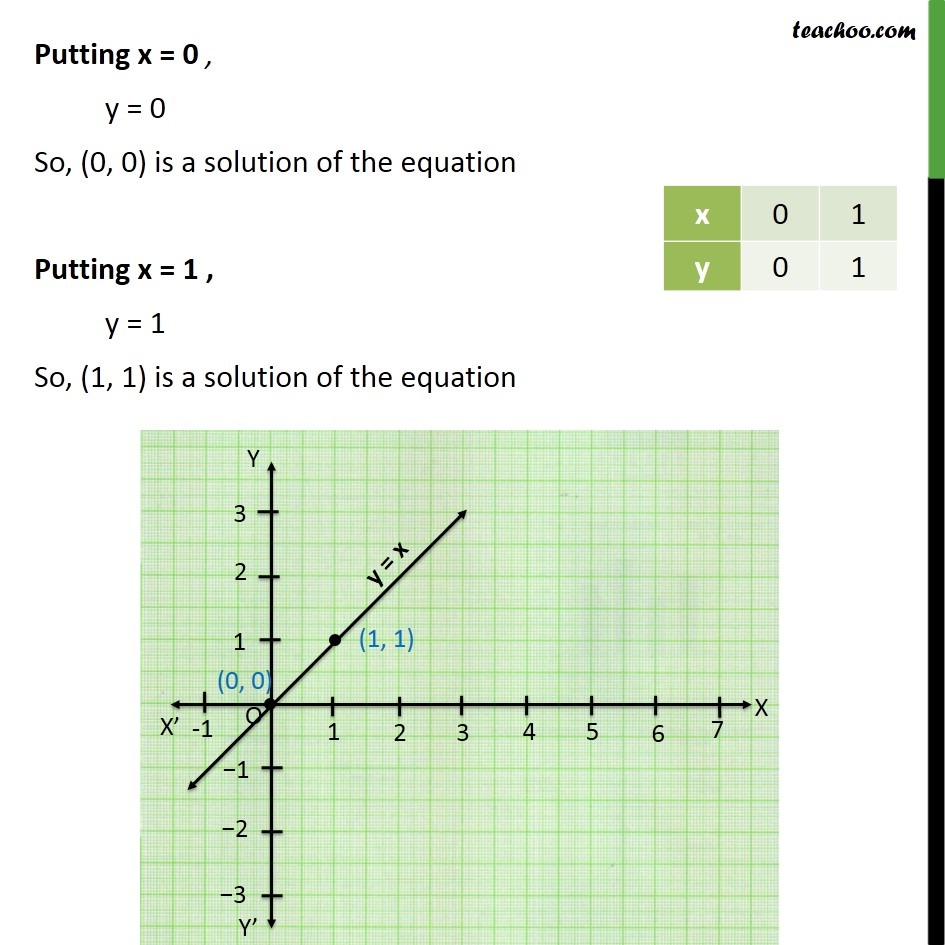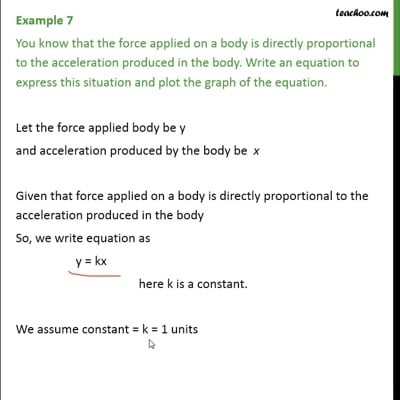Forming Equations and drawing graph

Chapter 4 Class 9 Linear Equations in Two Variables
Concept wiseThis video is only available for Teachoo black users

Introducing your new favourite teacher - Teachoo Black, at only ₹83 per month

### Transcript

Example 7 You know that the force applied on a body is directly proportional to the acceleration produced in the body. Write an equation to express this situation and plot the graph of the equation. Let the force applied body be y and acceleration produced by the body be x Given that force applied on a body is directly proportional to the acceleration produced in the body So, we write equation as y = kx here k is a constant. We assume constant = k = 1 units So, our equation becomes y = x We need to draw graph Putting x = 0 , y = 0 So, (0, 0) is a solution of the equation Putting x = 1 , y = 1 So, (1, 1) is a solution of the equation